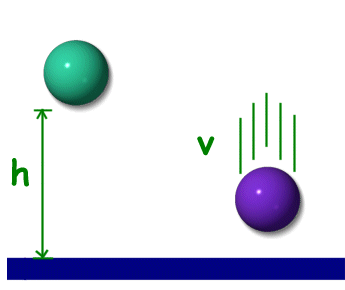Science >> Physics for Kids

# Physics for Kids

## Potential Energy

What is potential energy?

Potential energy is the stored energy an object has because of its position or state. A bicycle on top of a hill, a book held over your head, and a stretched spring all have potential energy.

How to Measure Potential Energy

The standard unit for measuring potential energy is the joule, which is abbreviated as "J."

How is it different from kinetic energy?

Potential energy is stored energy while kinetic energy is the energy of motion. When potential energy is used it is converted into kinetic energy. You can think of potential energy as kinetic energy waiting to happen.The green ball has potential energy due
to its height. The purple ball has kinetic
energy due to its velocity.

A Car on a Hill

We can compare potential and kinetic energy by considering a car on a hill. When the car is at the top of the hill it has the most potential energy. If it is sitting still, it has no kinetic energy. As the car begins to roll down the hill, it loses potential energy, but gains kinetic energy. The potential energy of the position of the car at the top of the hill is getting converted into kinetic energy.

Gravitational Potential Energy

One type of potential energy comes from the Earth's gravity. This is called gravitational potential energy (GPE). Gravitational potential energy is the energy stored in an object based on its height and mass. To calculate the gravitational potential energy we use the following equation:

GPE = mass * g * height
GPE = m*g*h

Where "g" is the standard acceleration of gravity which equals 9.8 m/s2. The height is determined based on the height the object could potentially fall. The height may be the distance above the ground or perhaps the lab table we are working on.

Example problems:

What is the potential energy of a 2 kg rock sitting at the top of a 10 meter high cliff?

GPE = mass * g * height
GPE = 2kg * 9.8 m/s2 * 10m
GPE = 196 J

Potential Energy and Work

The potential energy is equal to the amount of work done to get an object into its position. For example, if you were to lift a book off the floor and place it on a table. The potential energy of the book on the table will equal the amount of work it took to move the book from the floor to the table.

Other Types of Potential Energy
• Elastic - Elastic potential energy is stored when materials stretch or compress. Examples of elastic potential energy include springs, rubber bands, and slingshots.
• Electric - Electric potential energy is the capacity for doing work based on the object's electric charge.
• Nuclear - The potential energy of the particles inside an atom.
• Chemical - Chemical potential energy is the energy stored up in substances due to their chemical bonds. One example of this is the energy stored in gasoline for a car.
• Scottish scientist William Rankine first coined the term potential energy in the 19th century.
• The equation for calculating the potential energy of a spring is PE = 1/2 * k * x2, where k is the spring constant and x is the amount of compression.
• The concept of potential energy goes all the way back to Ancient Greece and the philosopher Aristotle.
Activities

More Physics Subjects on Motion, Work, and Energy

Science >> Physics for Kids